# RS Aggarwal Solutions for Class 9 Chapter 15: Volume and Surface Area of Solids Exercise 15B

## RS Aggarwal Solutions for Class 9 Maths Exercise 15B PDF

The subject experts at BYJU’S have prepared exercise wise solutions which are beneficial for students to obtain a good score in the exam. The students can obtain solutions in PDF format which can be downloaded effortlessly. The solutions are provided with step by step explanations in order to make the subject easy for students. RS Aggarwal Solutions are according to the CBSE guidelines to help students perform well in the board exam. The problems are solved with diagramatic representation in order to make them easy to understand. RS Aggarwal Solutions for Class 9 Maths Chapter 15 Volume and Surface Area of Solids Exercise 15B are provided here.

## RS Aggarwal Solutions for Class 9 Chapter 15: Volume and Surface Area of Solids Exercise 15B Download PDF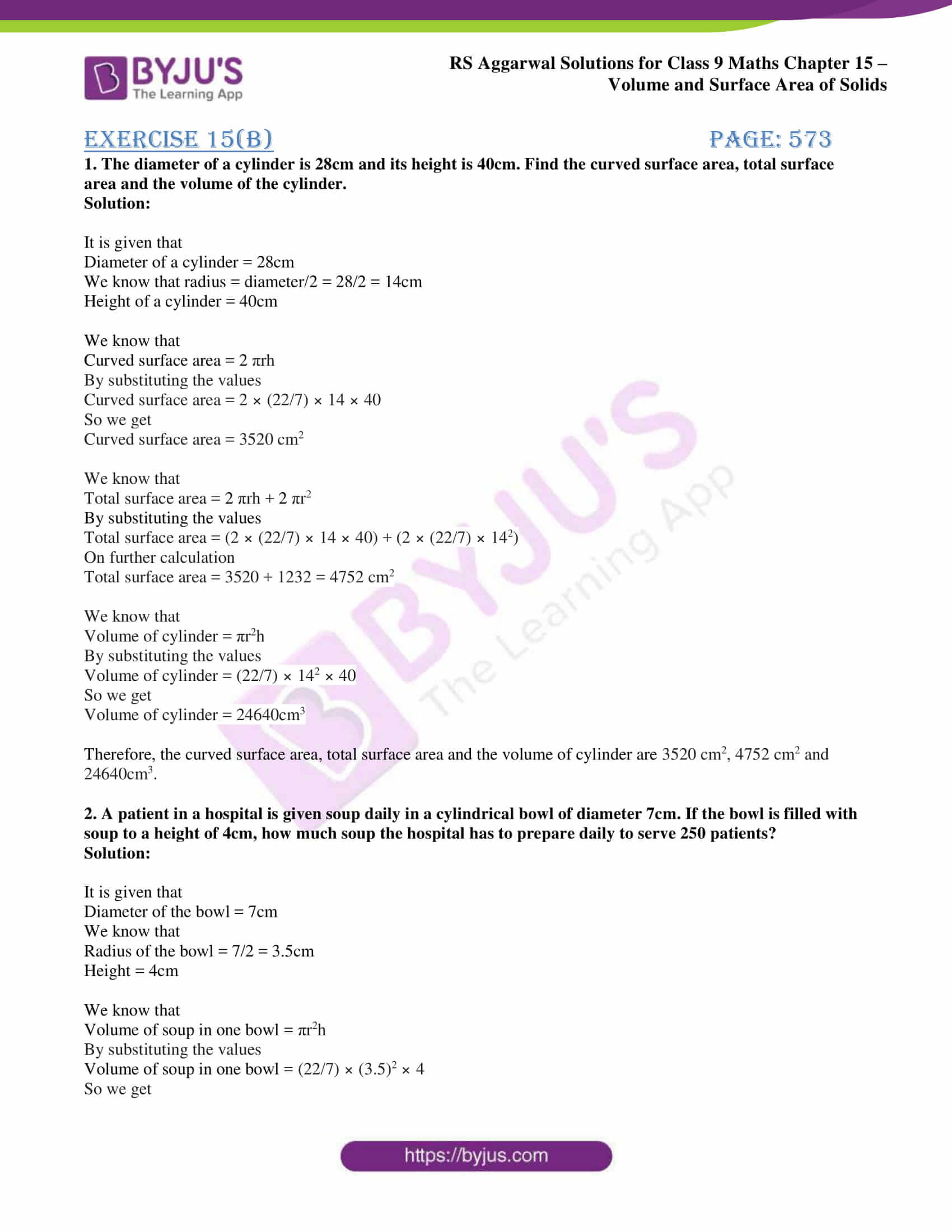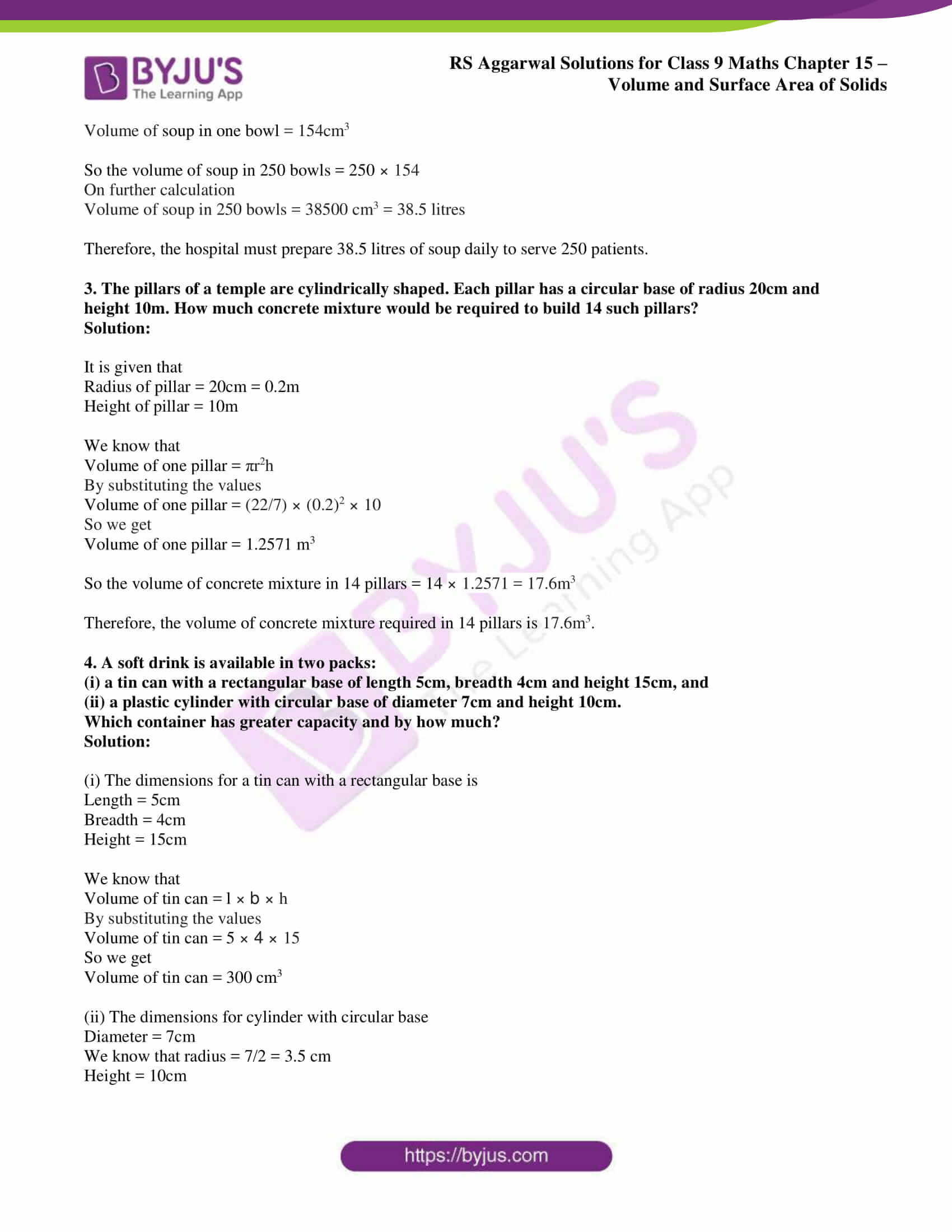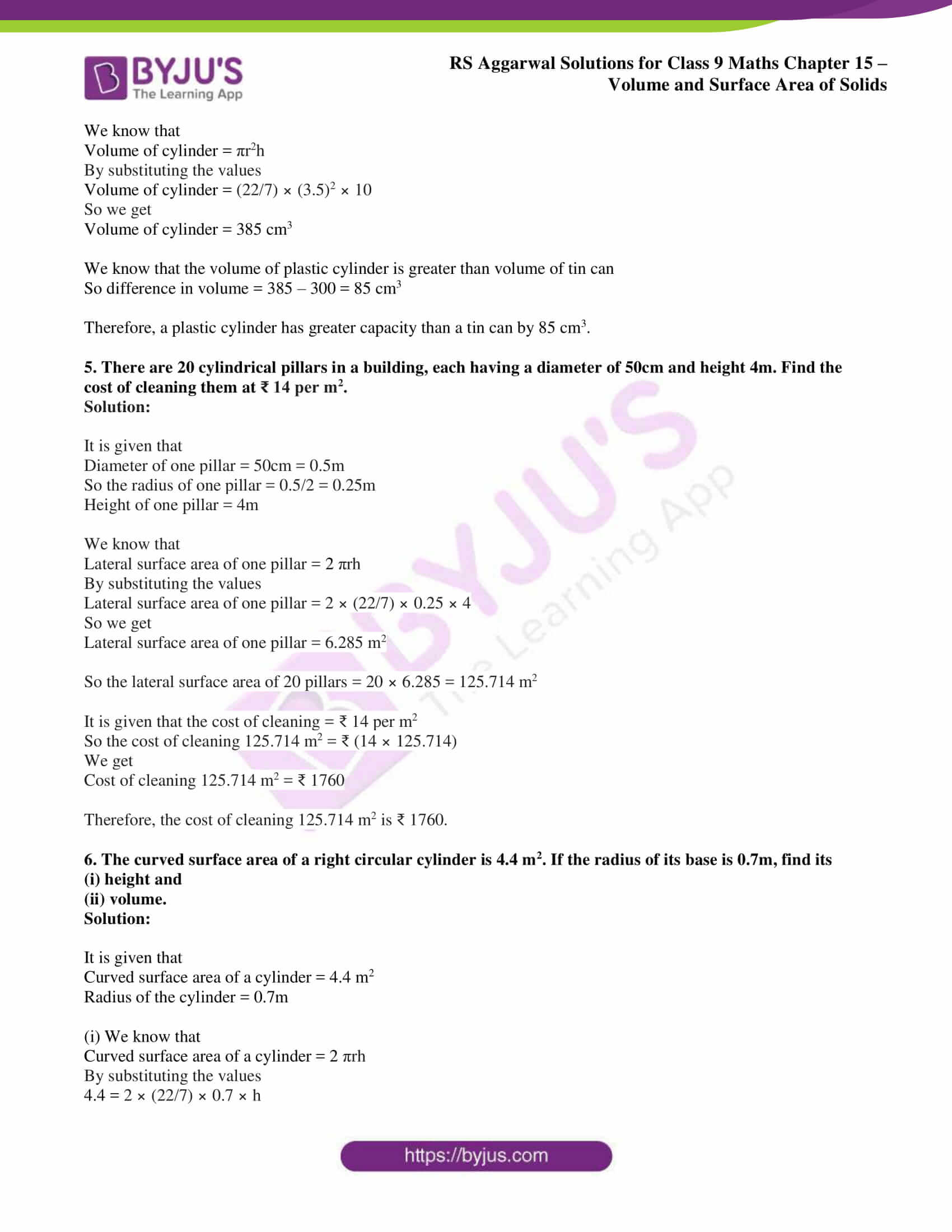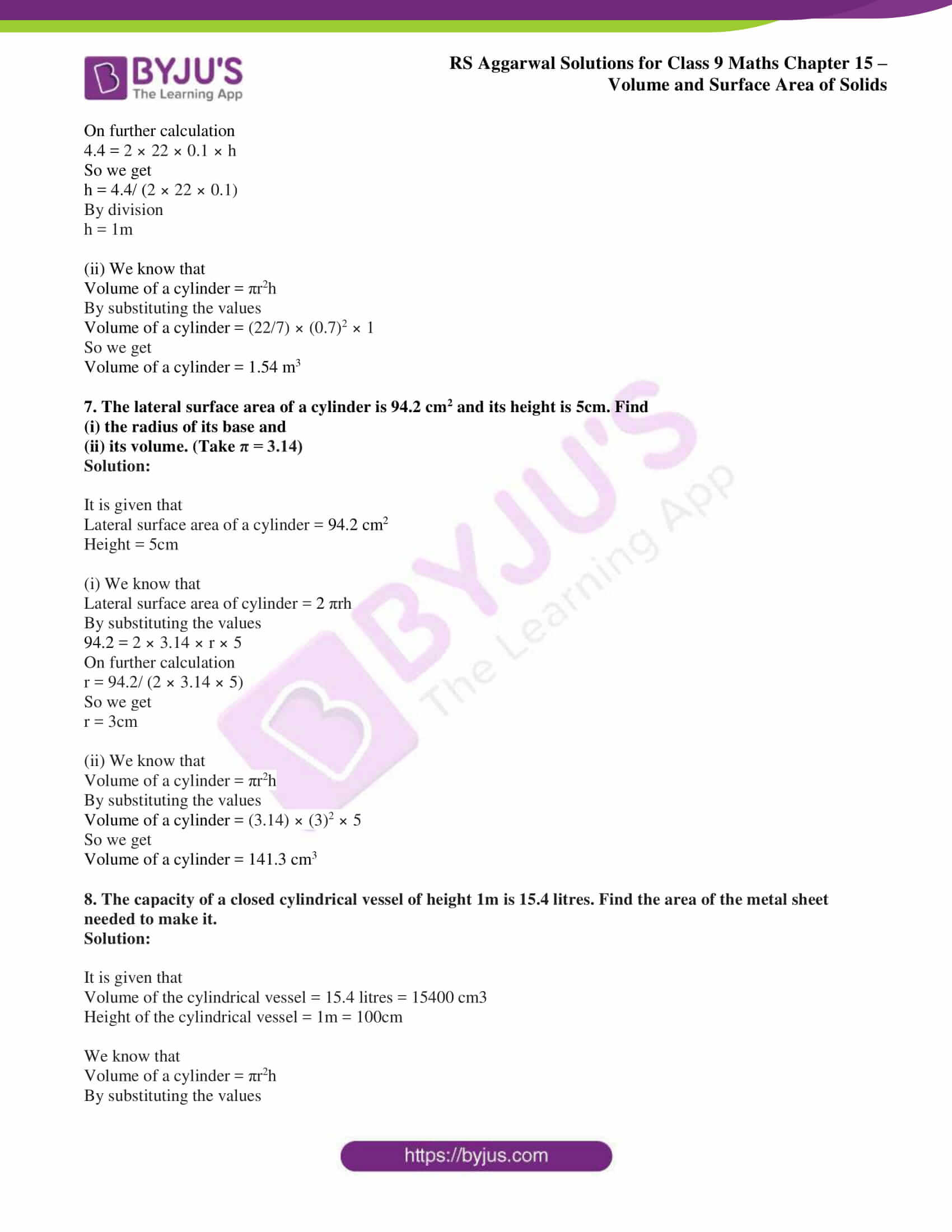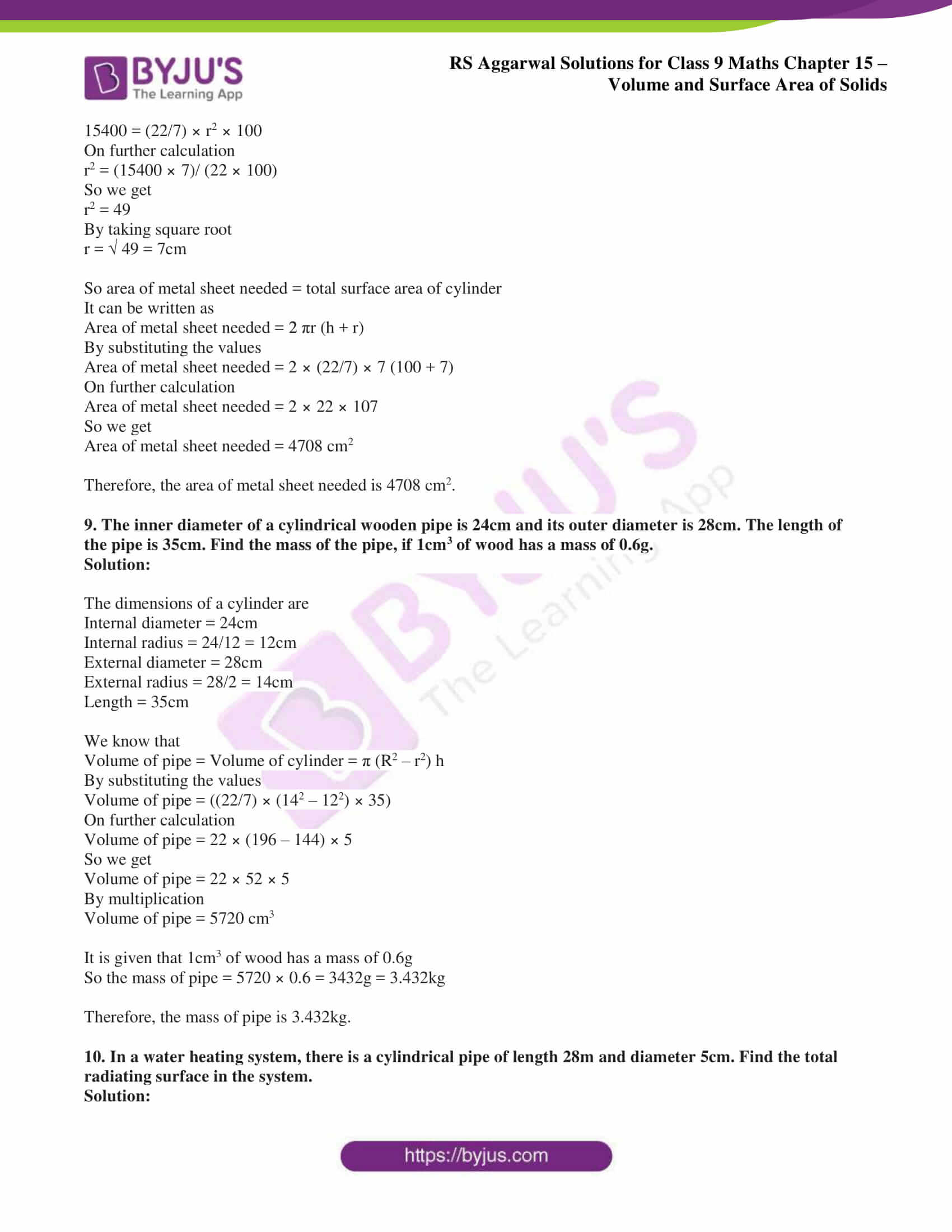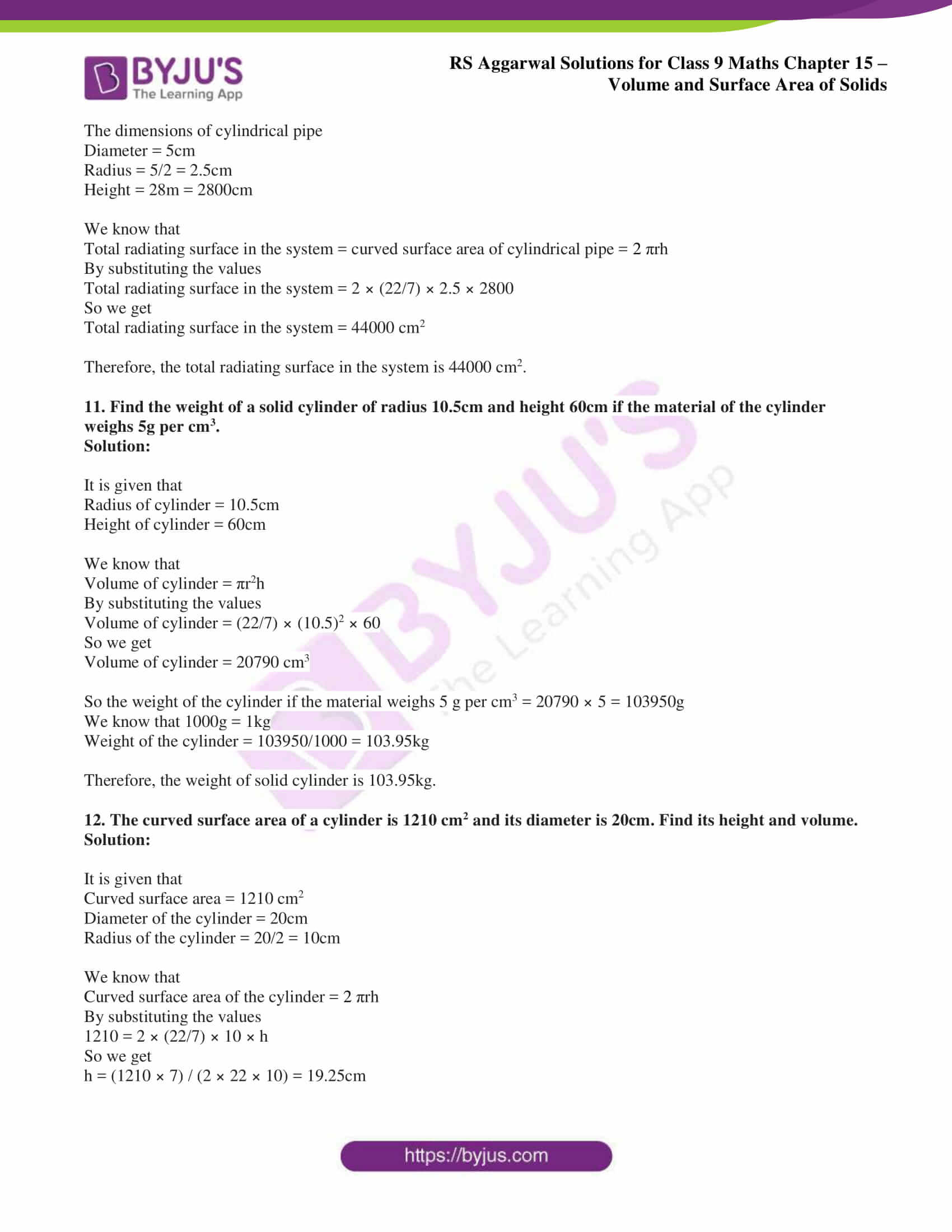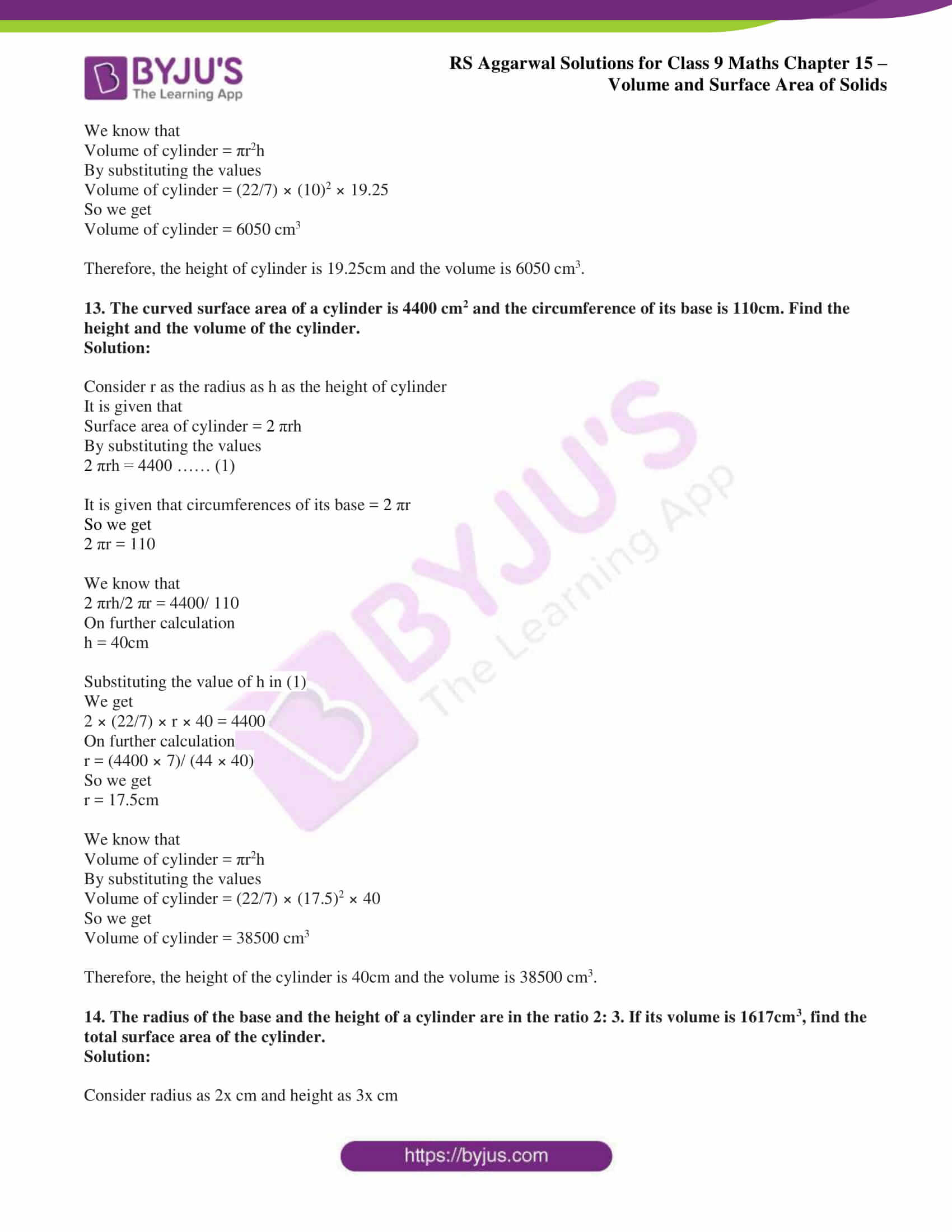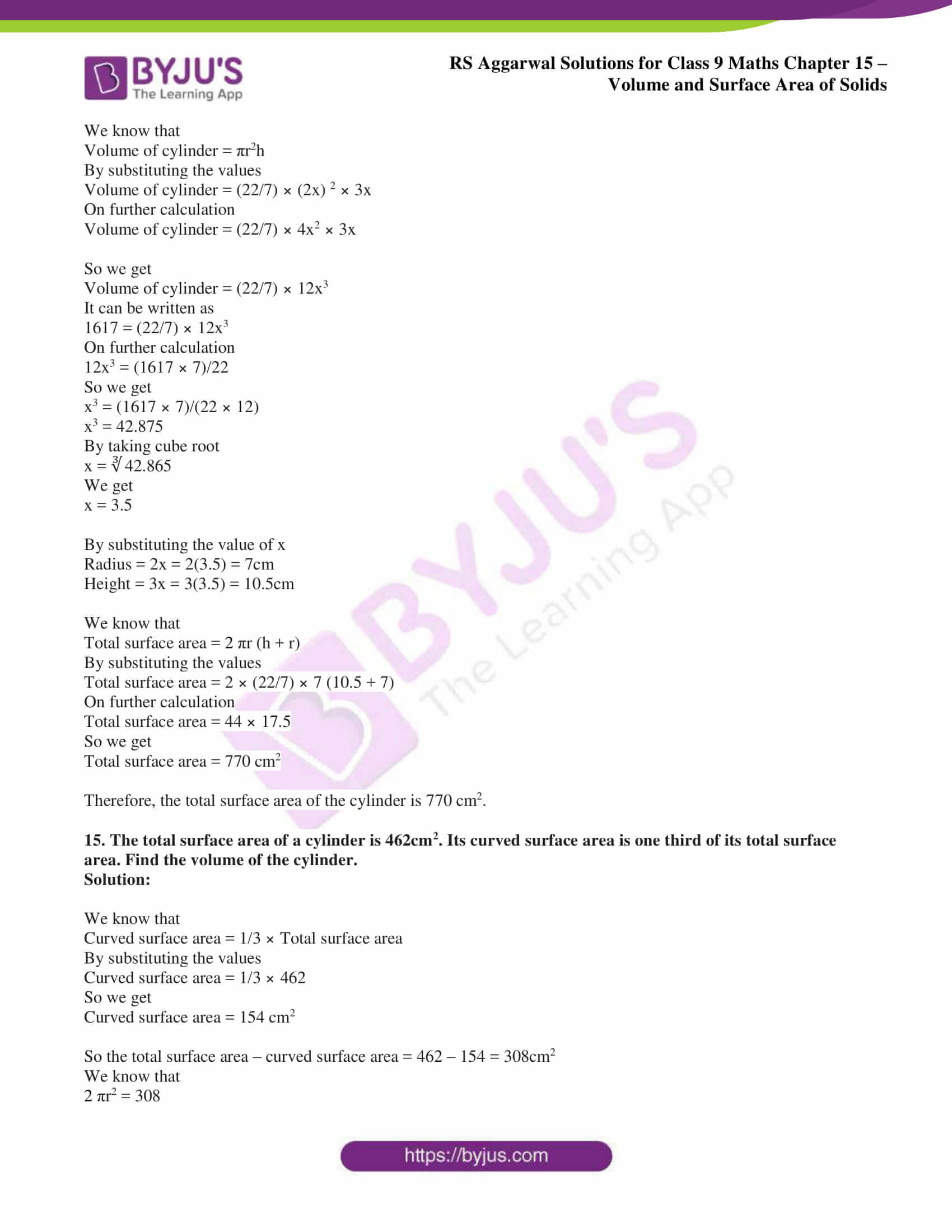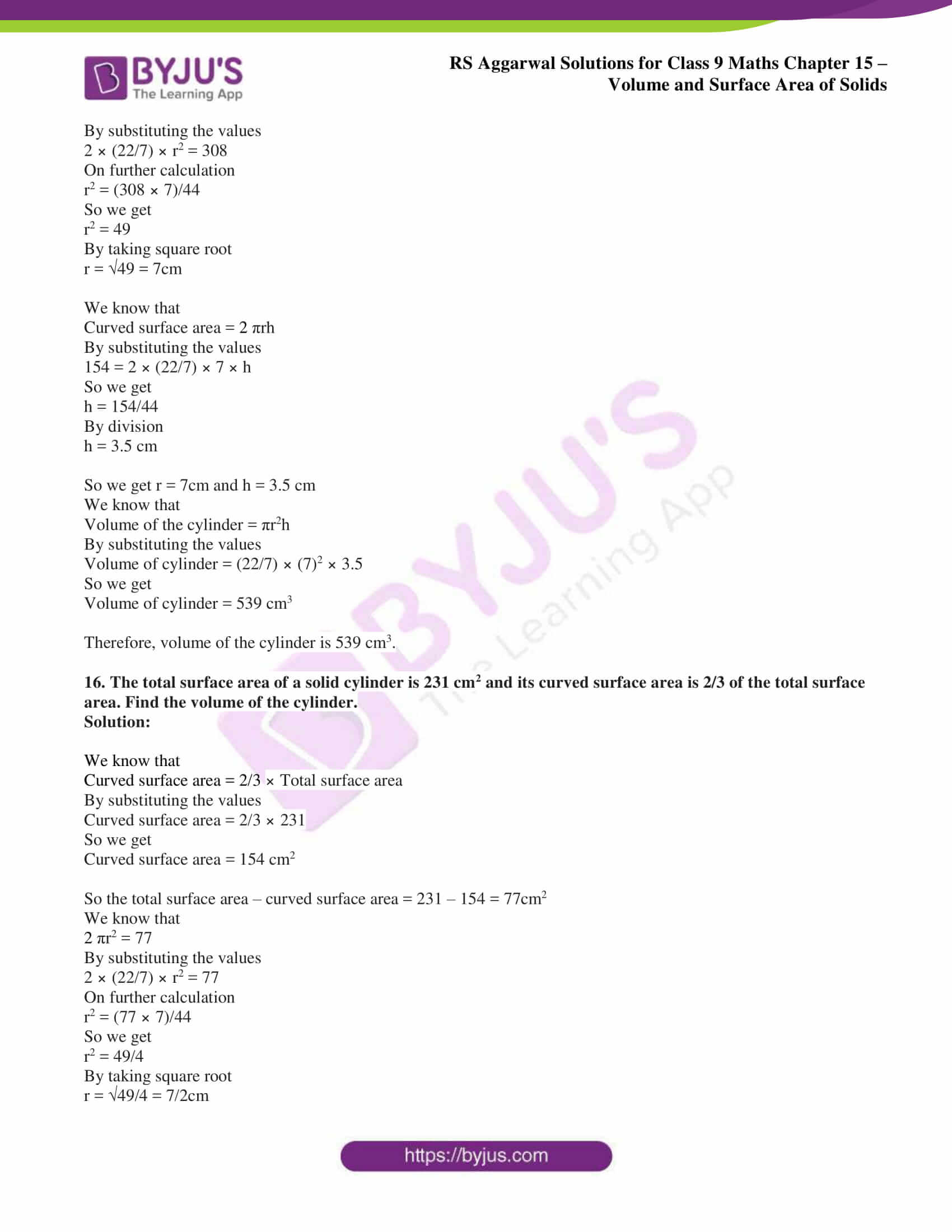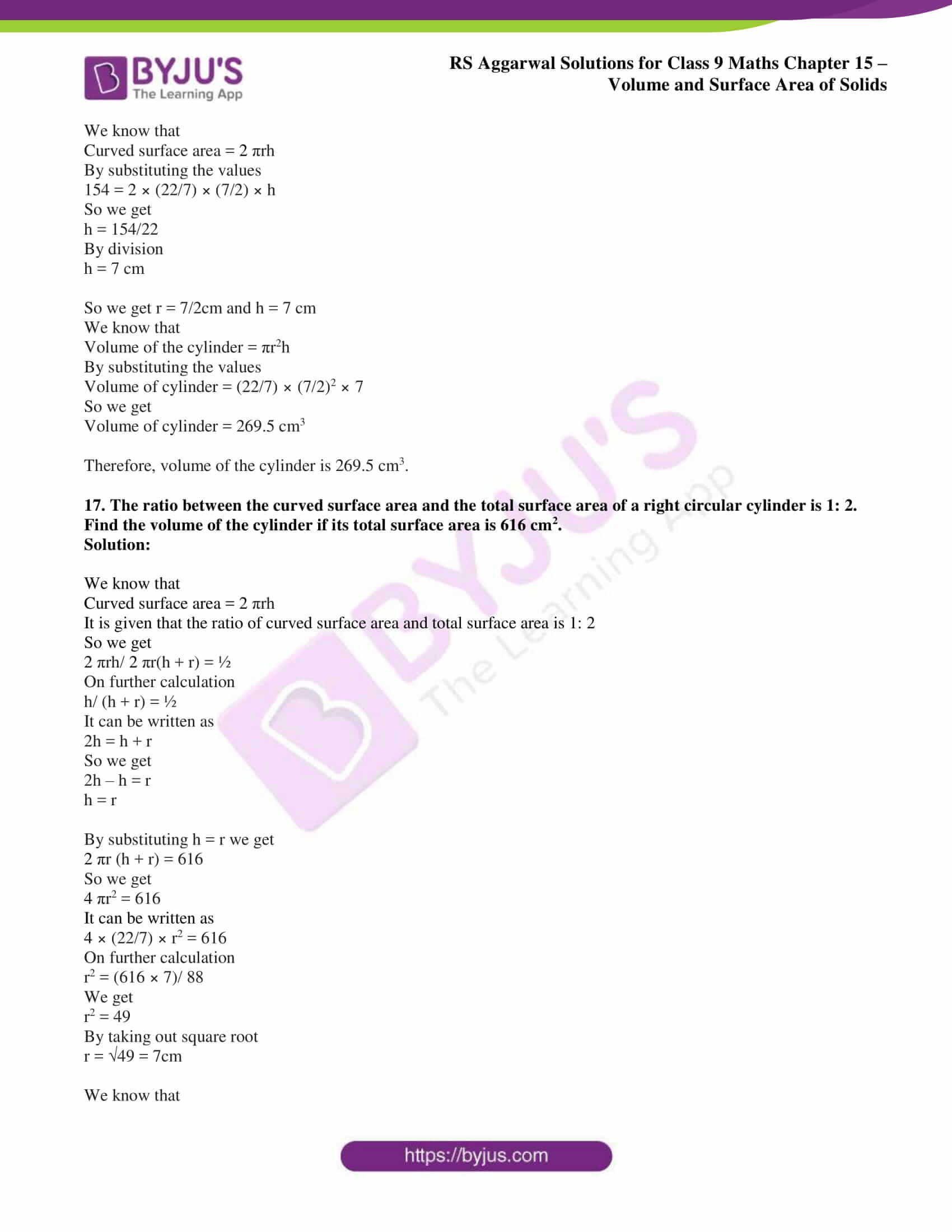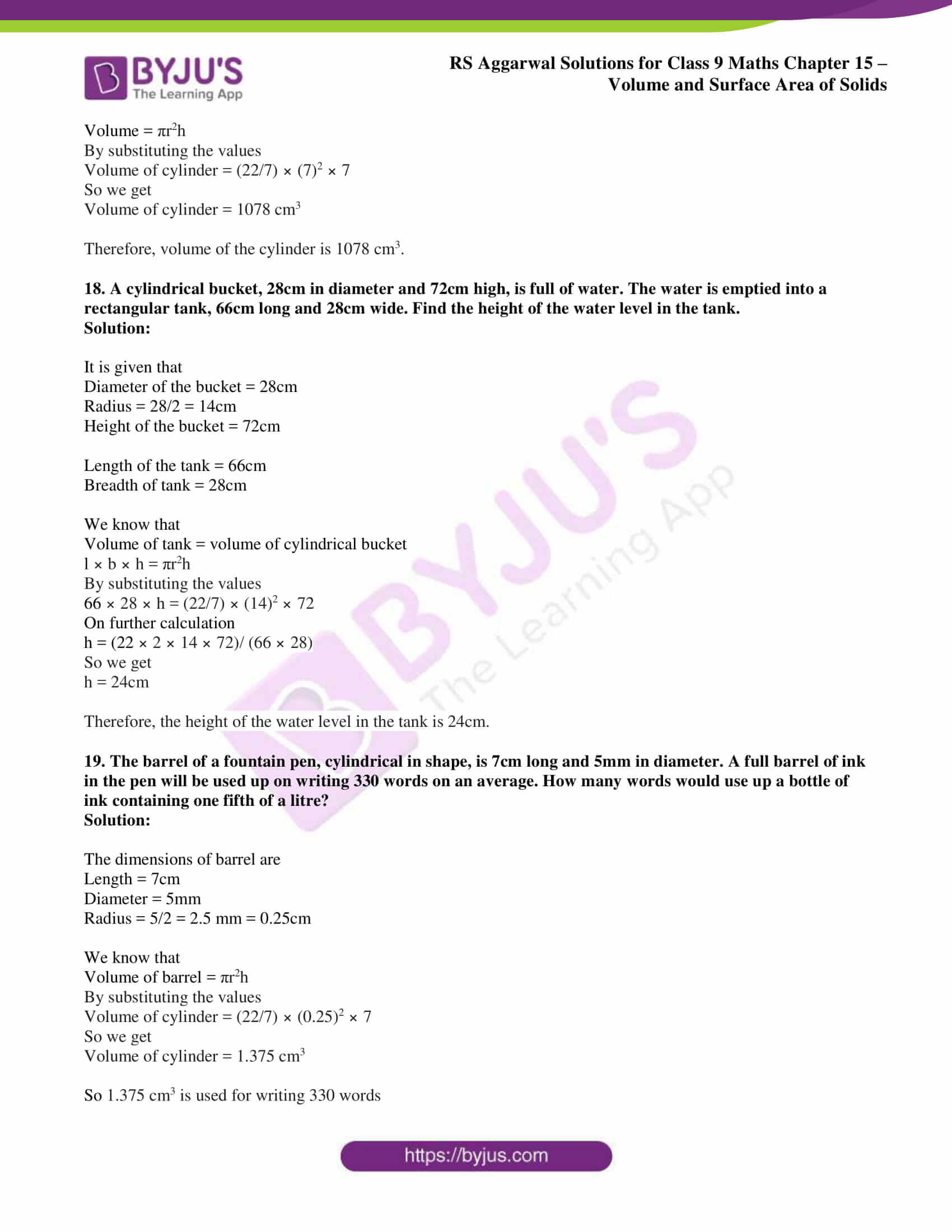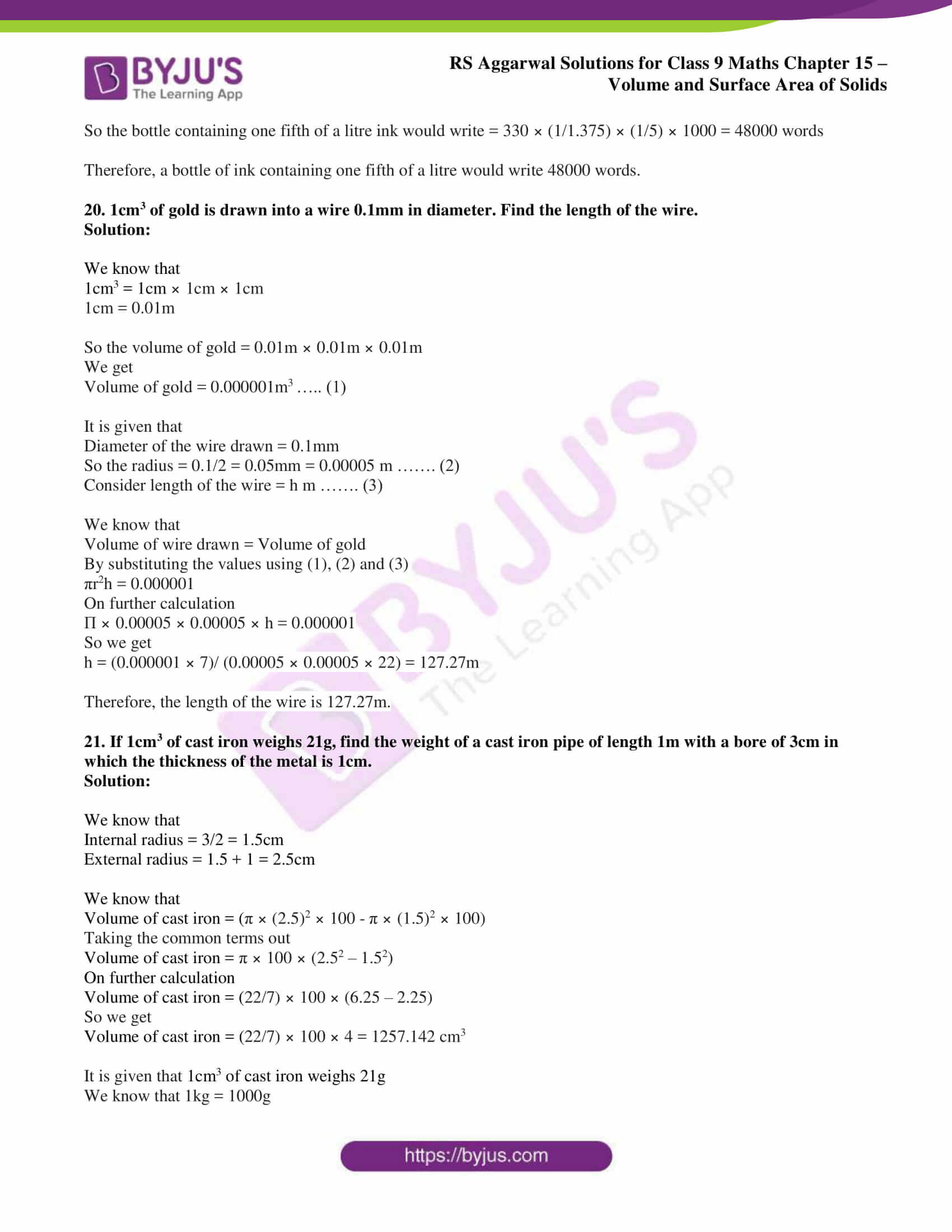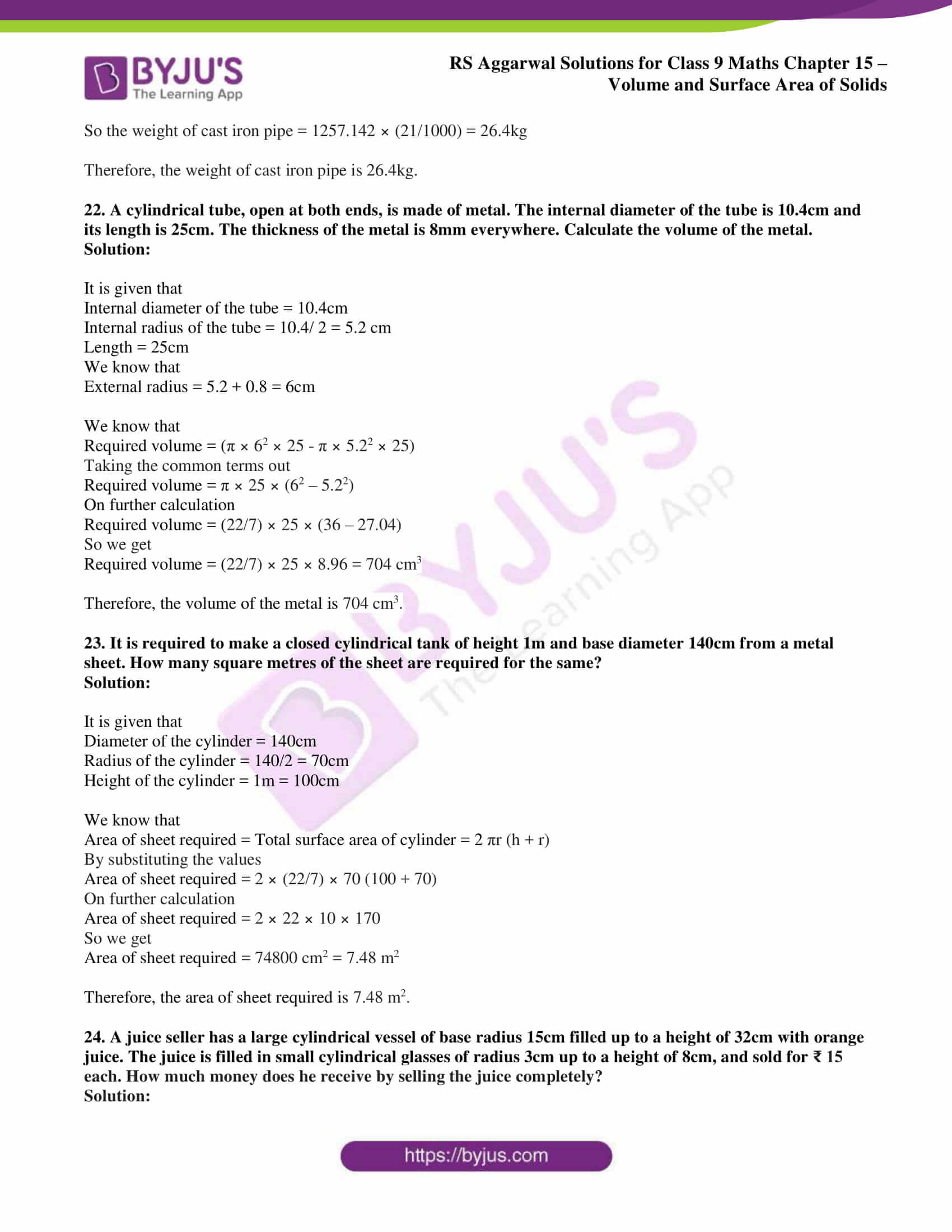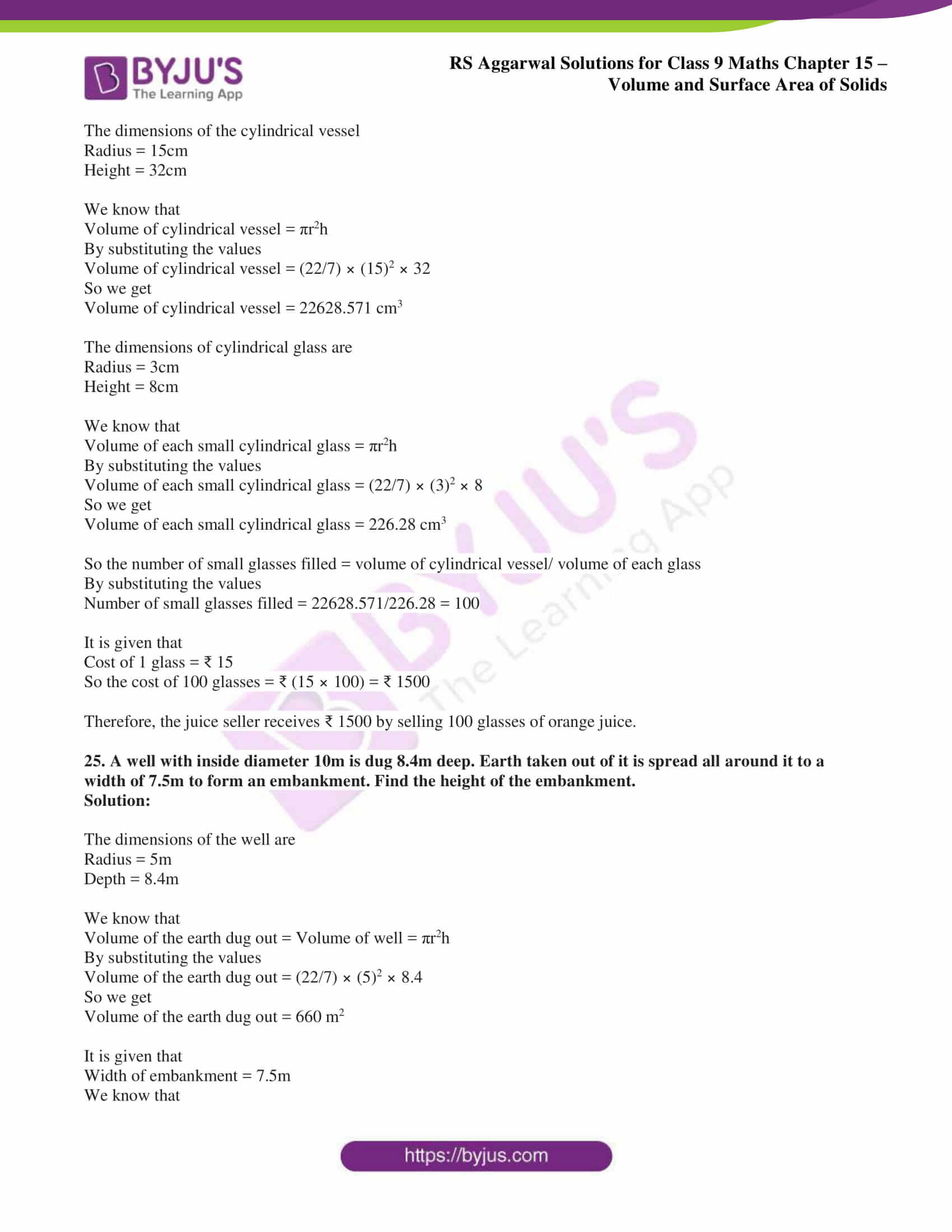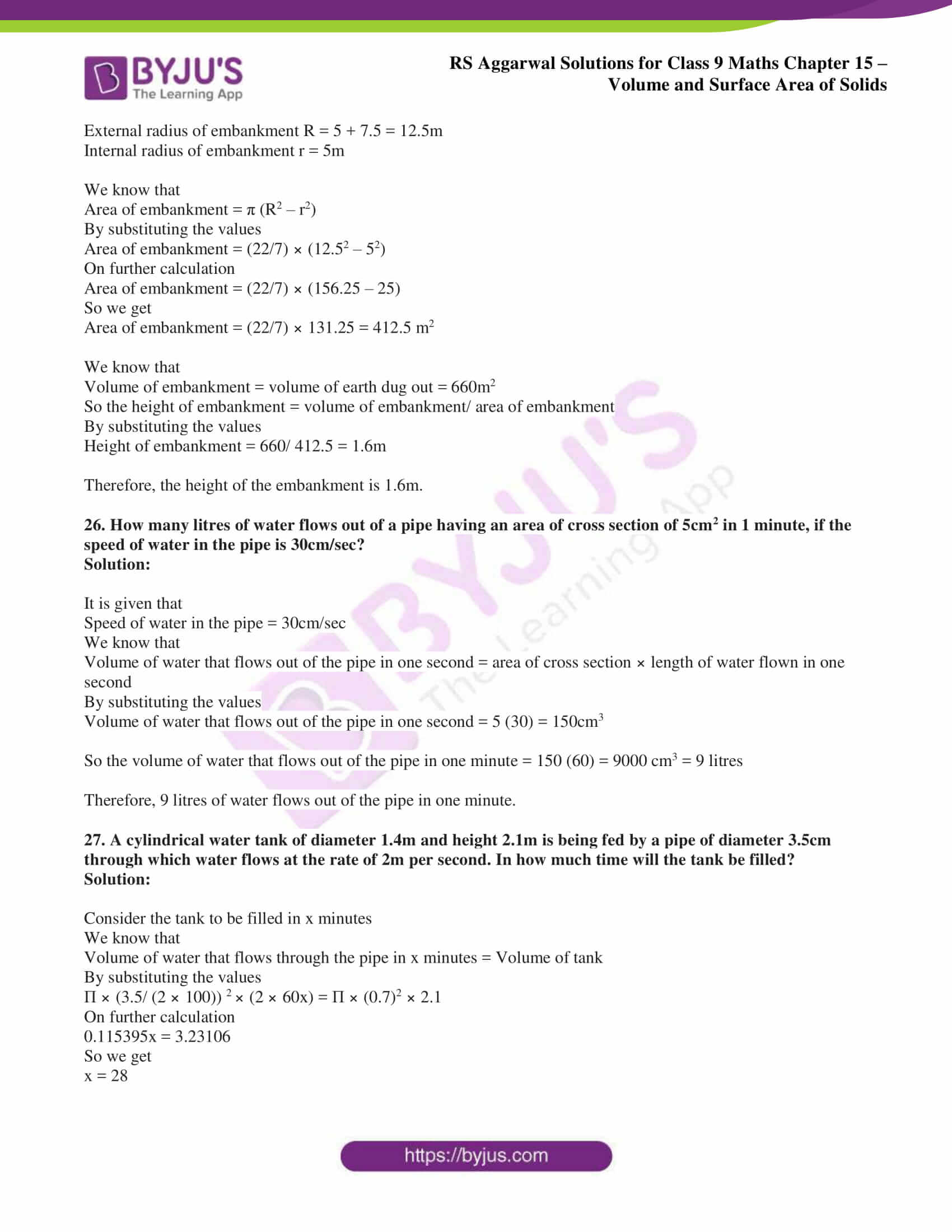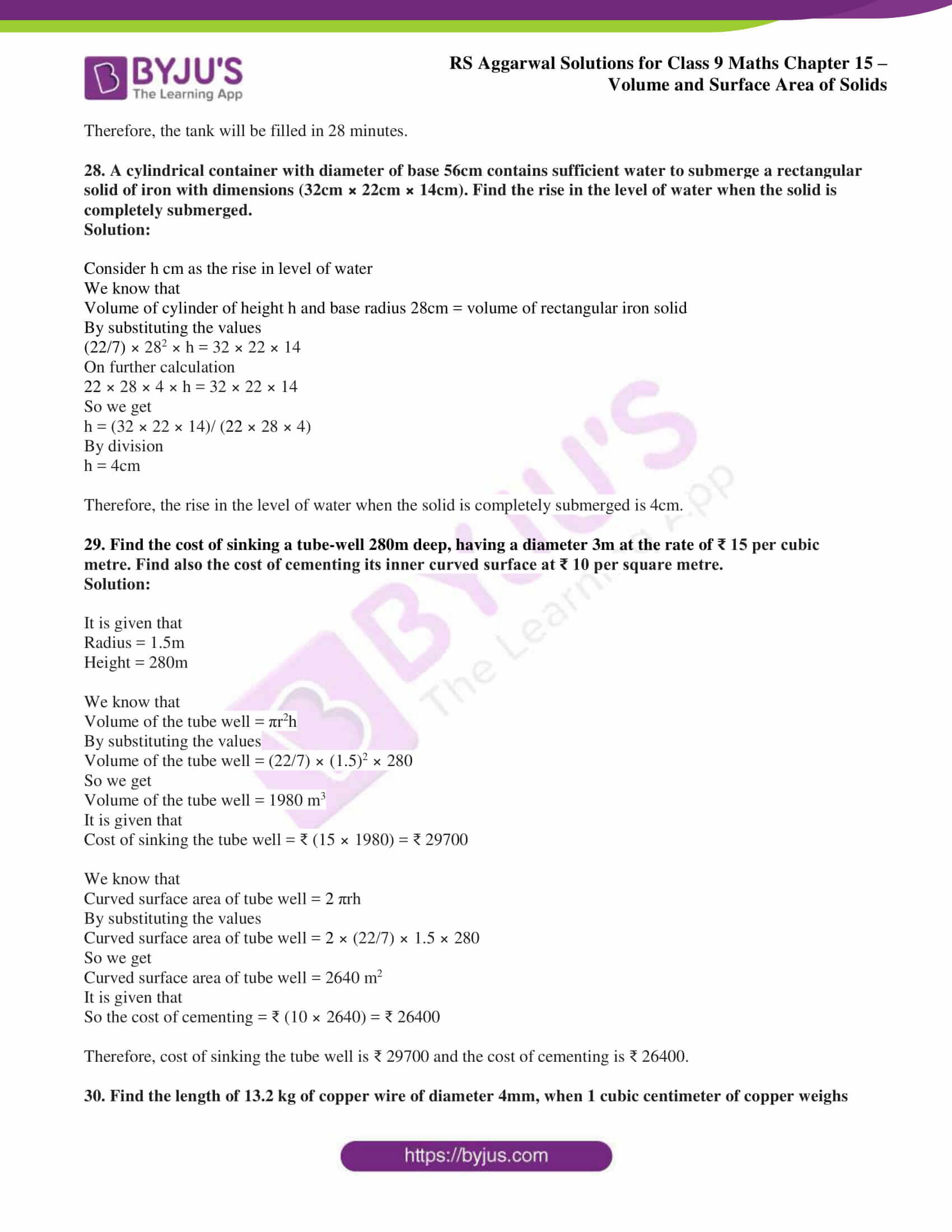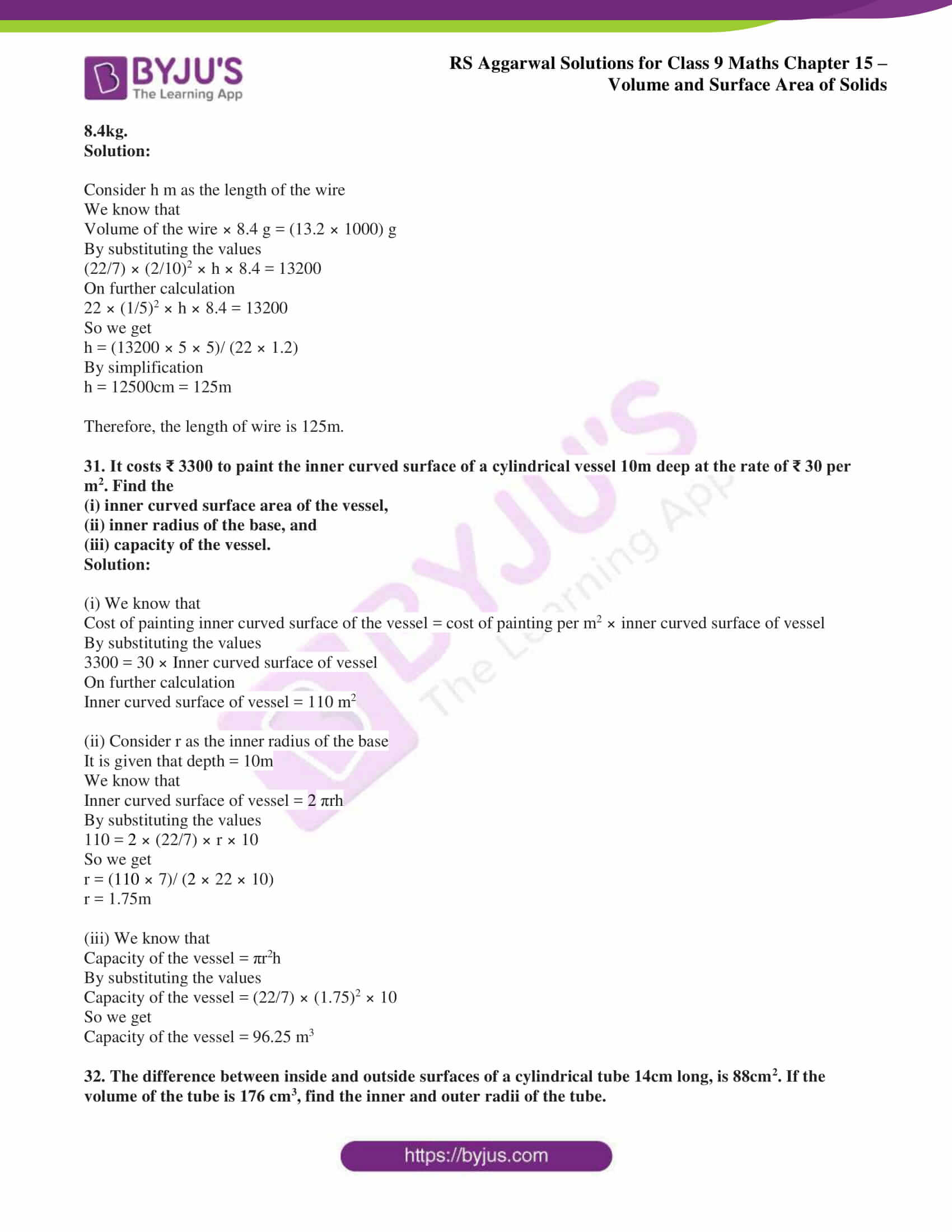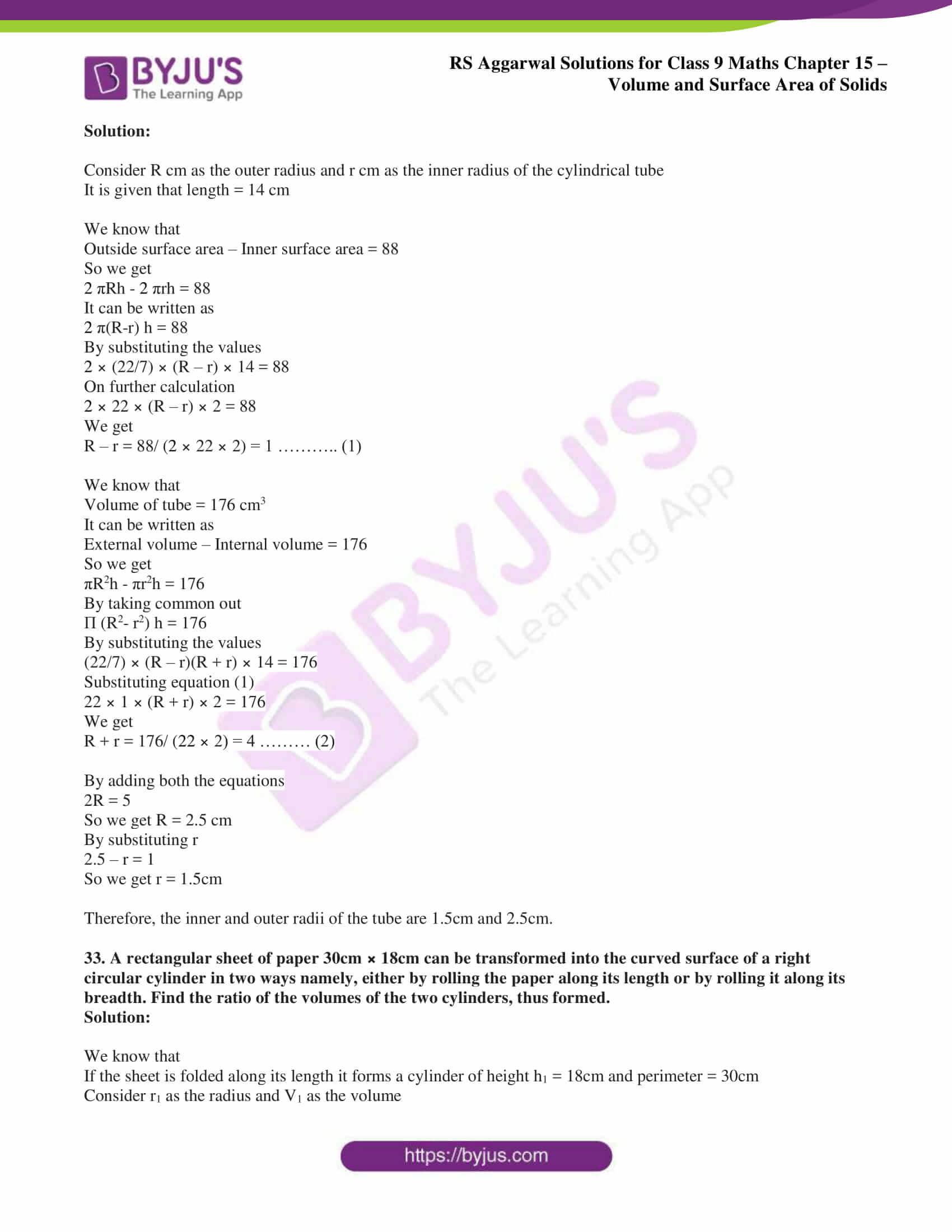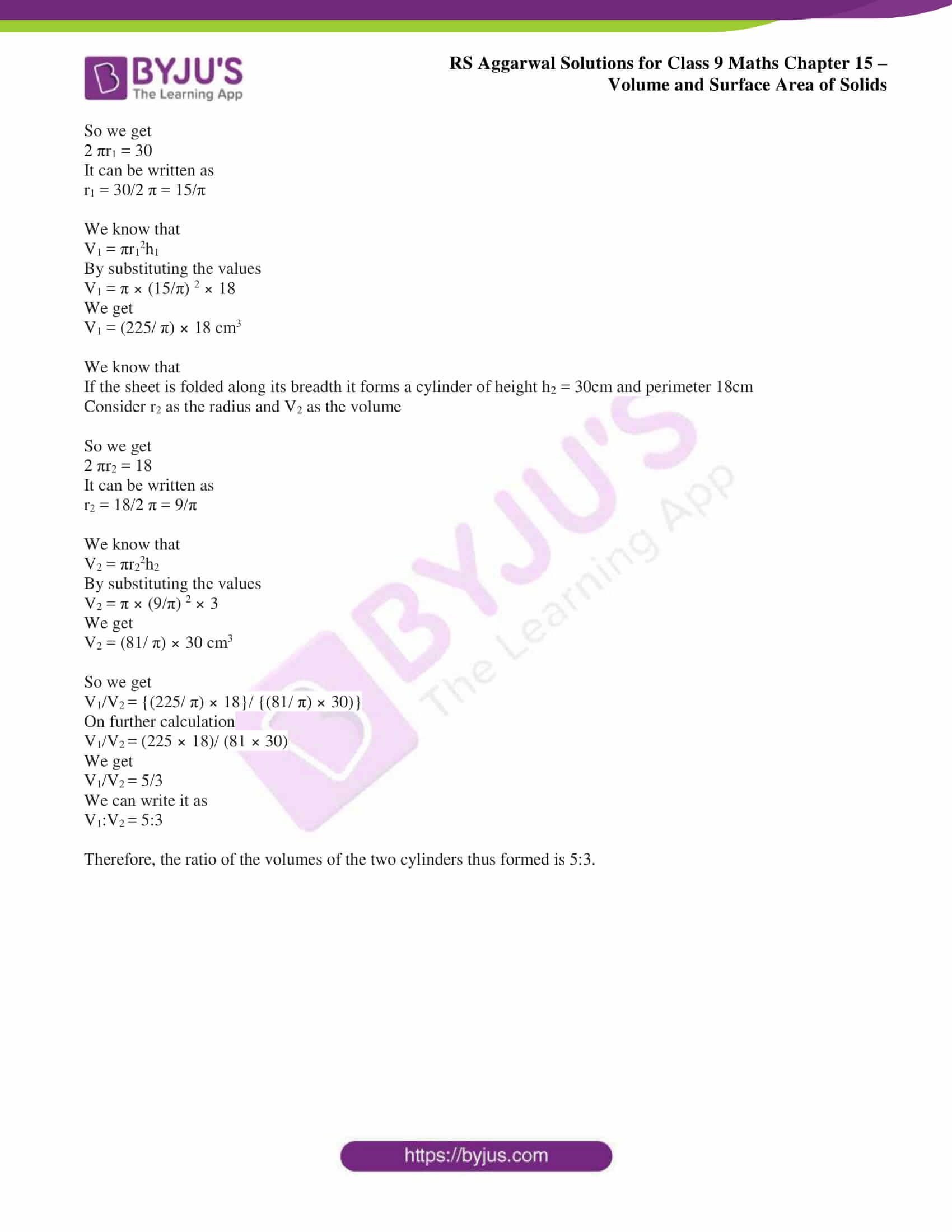## Access RS Aggarwal Solutions for Class 9 Chapter 15: Volumes and Surface Area of Solids Exercise 15B

1. The diameter of a cylinder is 28cm and its height is 40cm. Find the curved surface area, total surface area and the volume of the cylinder.

Solution:

It is given that

Diameter of a cylinder = 28cm

We know that radius = diameter/2 = 28/2 = 14cm

Height of a cylinder = 40cm

We know that

Curved surface area = 2 πrh

By substituting the values

Curved surface area = 2 × (22/7) × 14 × 40

So we get

Curved surface area = 3520 cm2

We know that

Total surface area = 2 πrh + 2 πr2

By substituting the values

Total surface area = (2 × (22/7) × 14 × 40) + (2 × (22/7) × 142)

On further calculation

Total surface area = 3520 + 1232 = 4752 cm2

We know that

Volume of cylinder = πr2h

By substituting the values

Volume of cylinder = (22/7) × 142 × 40

So we get

Volume of cylinder = 24640cm3

Therefore, the curved surface area, total surface area and the volume of cylinder are 3520 cm2, 4752 cm2 and 24640cm3.

2. A patient in a hospital is given soup daily in a cylindrical bowl of diameter 7cm. If the bowl is filled with soup to a height of 4cm, how much soup the hospital has to prepare daily to serve 250 patients?

Solution:

It is given that

Diameter of the bowl = 7cm

We know that

Radius of the bowl = 7/2 = 3.5cm

Height = 4cm

We know that

Volume of soup in one bowl = πr2h

By substituting the values

Volume of soup in one bowl = (22/7) × (3.5)2 × 4

So we get

Volume of soup in one bowl = 154cm3

So the volume of soup in 250 bowls = 250 × 154

On further calculation

Volume of soup in 250 bowls = 38500 cm3 = 38.5 litres

Therefore, the hospital must prepare 38.5 litres of soup daily to serve 250 patients.

3. The pillars of a temple are cylindrically shaped. Each pillar has a circular base of radius 20cm and height 10m. How much concrete mixture would be required to build 14 such pillars?

Solution:

It is given that

Radius of pillar = 20cm = 0.2m

Height of pillar = 10m

We know that

Volume of one pillar = πr2h

By substituting the values

Volume of one pillar = (22/7) × (0.2)2 × 10

So we get

Volume of one pillar = 1.2571 m3

So the volume of concrete mixture in 14 pillars = 14 × 1.2571 = 17.6m3

Therefore, the volume of concrete mixture required in 14 pillars is 17.6m3.

4. A soft drink is available in two packs:

(i) a tin can with a rectangular base of length 5cm, breadth 4cm and height 15cm, and

(ii) a plastic cylinder with circular base of diameter 7cm and height 10cm.

Which container has greater capacity and by how much?

Solution:

(i) The dimensions for a tin can with a rectangular base is

Length = 5cm

Height = 15cm

We know that

Volume of tin can = l × b × h

By substituting the values

Volume of tin can = 5 × 4 × 15

So we get

Volume of tin can = 300 cm3

(ii) The dimensions for cylinder with circular base

Diameter = 7cm

We know that radius = 7/2 = 3.5 cm

Height = 10cm

We know that

Volume of cylinder = πr2h

By substituting the values

Volume of cylinder = (22/7) × (3.5)2 × 10

So we get

Volume of cylinder = 385 cm3

We know that the volume of plastic cylinder is greater than volume of tin can

So difference in volume = 385 – 300 = 85 cm3

Therefore, a plastic cylinder has greater capacity than a tin can by 85 cm3.

5. There are 20 cylindrical pillars in a building, each having a diameter of 50cm and height 4m. Find the cost of cleaning them at ₹ 14 per m2.

Solution:

It is given that

Diameter of one pillar = 50cm = 0.5m

So the radius of one pillar = 0.5/2 = 0.25m

Height of one pillar = 4m

We know that

Lateral surface area of one pillar = 2 πrh

By substituting the values

Lateral surface area of one pillar = 2 × (22/7) × 0.25 × 4

So we get

Lateral surface area of one pillar = 6.285 m2

So the lateral surface area of 20 pillars = 20 × 6.285 = 125.714 m2

It is given that the cost of cleaning = ₹ 14 per m2

So the cost of cleaning 125.714 m2 = ₹ (14 × 125.714)

We get

Cost of cleaning 125.714 m2 = ₹ 1760

Therefore, the cost of cleaning 125.714 m2 is ₹ 1760.

6. The curved surface area of a right circular cylinder is 4.4 m2. If the radius of its base is 0.7m, find its

(i) height and

(ii) volume.

Solution:

It is given that

Curved surface area of a cylinder = 4.4 m2

Radius of the cylinder = 0.7m

(i) We know that

Curved surface area of a cylinder = 2 πrh

By substituting the values

4.4 = 2 × (22/7) × 0.7 × h

On further calculation

4.4 = 2 × 22 × 0.1 × h

So we get

h = 4.4/ (2 × 22 × 0.1)

By division

h = 1m

(ii) We know that

Volume of a cylinder = πr2h

By substituting the values

Volume of a cylinder = (22/7) × (0.7)2 × 1

So we get

Volume of a cylinder = 1.54 m3

7. The lateral surface area of a cylinder is 94.2 cm2 and its height is 5cm. Find

(i) the radius of its base and

(ii) its volume. (Take π = 3.14)

Solution:

It is given that

Lateral surface area of a cylinder = 94.2 cm2

Height = 5cm

(i) We know that

Lateral surface area of cylinder = 2 πrh

By substituting the values

94.2 = 2 × 3.14 × r × 5

On further calculation

r = 94.2/ (2 × 3.14 × 5)

So we get

r = 3cm

(ii) We know that

Volume of a cylinder = πr2h

By substituting the values

Volume of a cylinder = (3.14) × (3)2 × 5

So we get

Volume of a cylinder = 141.3 cm3

8. The capacity of a closed cylindrical vessel of height 1m is 15.4 litres. Find the area of the metal sheet needed to make it.

Solution:

It is given that

Volume of the cylindrical vessel = 15.4 litres = 15400 cm3

Height of the cylindrical vessel = 1m = 100cm

We know that

Volume of a cylinder = πr2h

By substituting the values

15400 = (22/7) × r2 × 100

On further calculation

r2 = (15400 × 7)/ (22 × 100)

So we get

r2 = 49

By taking square root

r = √ 49 = 7cm

So area of metal sheet needed = total surface area of cylinder

It can be written as

Area of metal sheet needed = 2 πr (h + r)

By substituting the values

Area of metal sheet needed = 2 × (22/7) × 7 (100 + 7)

On further calculation

Area of metal sheet needed = 2 × 22 × 107

So we get

Area of metal sheet needed = 4708 cm2

Therefore, the area of metal sheet needed is 4708 cm2.

9. The inner diameter of a cylindrical wooden pipe is 24cm and its outer diameter is 28cm. The length of the pipe is 35cm. Find the mass of the pipe, if 1cm3 of wood has a mass of 0.6g.

Solution:

The dimensions of a cylinder are

Internal diameter = 24cm

Internal radius = 24/12 = 12cm

External diameter = 28cm

External radius = 28/2 = 14cm

Length = 35cm

We know that

Volume of pipe = Volume of cylinder = π (R2 – r2) h

By substituting the values

Volume of pipe = ((22/7) × (142 – 122) × 35)

On further calculation

Volume of pipe = 22 × (196 – 144) × 5

So we get

Volume of pipe = 22 × 52 × 5

By multiplication

Volume of pipe = 5720 cm3

It is given that 1cm3 of wood has a mass of 0.6g

So the mass of pipe = 5720 × 0.6 = 3432g = 3.432kg

Therefore, the mass of pipe is 3.432kg.

10. In a water heating system, there is a cylindrical pipe of length 28m and diameter 5cm. Find the total radiating surface in the system.

Solution:

The dimensions of cylindrical pipe

Diameter = 5cm

Height = 28m = 2800cm

We know that

Total radiating surface in the system = curved surface area of cylindrical pipe = 2 πrh

By substituting the values

Total radiating surface in the system = 2 × (22/7) × 2.5 × 2800

So we get

Total radiating surface in the system = 44000 cm2

Therefore, the total radiating surface in the system is 44000 cm2.

11. Find the weight of a solid cylinder of radius 10.5cm and height 60cm if the material of the cylinder weighs 5g per cm3.

Solution:

It is given that

Height of cylinder = 60cm

We know that

Volume of cylinder = πr2h

By substituting the values

Volume of cylinder = (22/7) × (10.5)2 × 60

So we get

Volume of cylinder = 20790 cm3

So the weight of the cylinder if the material weighs 5 g per cm3 = 20790 × 5 = 103950g

We know that 1000g = 1kg

Weight of the cylinder = 103950/1000 = 103.95kg

Therefore, the weight of solid cylinder is 103.95kg.

12. The curved surface area of a cylinder is 1210 cm2 and its diameter is 20cm. Find its height and volume.

Solution:

It is given that

Curved surface area = 1210 cm2

Diameter of the cylinder = 20cm

Radius of the cylinder = 20/2 = 10cm

We know that

Curved surface area of the cylinder = 2 πrh

By substituting the values

1210 = 2 × (22/7) × 10 × h

So we get

h = (1210 × 7) / (2 × 22 × 10) = 19.25cm

We know that

Volume of cylinder = πr2h

By substituting the values

Volume of cylinder = (22/7) × (10)2 × 19.25

So we get

Volume of cylinder = 6050 cm3

Therefore, the height of cylinder is 19.25cm and the volume is 6050 cm3.

13. The curved surface area of a cylinder is 4400 cm2 and the circumference of its base is 110cm. Find the height and the volume of the cylinder.

Solution:

Consider r as the radius as h as the height of cylinder

It is given that

Surface area of cylinder = 2 πrh

By substituting the values

2 πrh = 4400 …… (1)

It is given that circumferences of its base = 2 πr

So we get

2 πr = 110

We know that

2 πrh/2 πr = 4400/ 110

On further calculation

h = 40cm

Substituting the value of h in (1)

We get

2 × (22/7) × r × 40 = 4400

On further calculation

r = (4400 × 7)/ (44 × 40)

So we get

r = 17.5cm

We know that

Volume of cylinder = πr2h

By substituting the values

Volume of cylinder = (22/7) × (17.5)2 × 40

So we get

Volume of cylinder = 38500 cm3

Therefore, the height of the cylinder is 40cm and the volume is 38500 cm3.

14. The radius of the base and the height of a cylinder are in the ratio 2: 3. If its volume is 1617cm3, find the total surface area of the cylinder.

Solution:

Consider radius as 2x cm and height as 3x cm

We know that

Volume of cylinder = πr2h

By substituting the values

Volume of cylinder = (22/7) × (2x) 2 × 3x

On further calculation

Volume of cylinder = (22/7) × 4x2 × 3x

So we get

Volume of cylinder = (22/7) × 12x3

It can be written as

1617 = (22/7) × 12x3

On further calculation

12x3 = (1617 × 7)/22

So we get

x3 = (1617 × 7)/(22 × 12)

x3 = 42.875

By taking cube root

x = ∛ 42.865

We get

x = 3.5

By substituting the value of x

Radius = 2x = 2(3.5) = 7cm

Height = 3x = 3(3.5) = 10.5cm

We know that

Total surface area = 2 πr (h + r)

By substituting the values

Total surface area = 2 × (22/7) × 7 (10.5 + 7)

On further calculation

Total surface area = 44 × 17.5

So we get

Total surface area = 770 cm2

Therefore, the total surface area of the cylinder is 770 cm2.

15. The total surface area of a cylinder is 462cm2. Its curved surface area is one third of its total surface area. Find the volume of the cylinder.

Solution:

We know that

Curved surface area = 1/3 × Total surface area

By substituting the values

Curved surface area = 1/3 × 462

So we get

Curved surface area = 154 cm2

So the total surface area – curved surface area = 462 – 154 = 308cm2

We know that

2 πr2 = 308

By substituting the values

2 × (22/7) × r2 = 308

On further calculation

r2 = (308 × 7)/44

So we get

r2 = 49

By taking square root

r = √49 = 7cm

We know that

Curved surface area = 2 πrh

By substituting the values

154 = 2 × (22/7) × 7 × h

So we get

h = 154/44

By division

h = 3.5 cm

So we get r = 7cm and h = 3.5 cm

We know that

Volume of the cylinder = πr2h

By substituting the values

Volume of cylinder = (22/7) × (7)2 × 3.5

So we get

Volume of cylinder = 539 cm3

Therefore, volume of the cylinder is 539 cm3.

16. The total surface area of a solid cylinder is 231 cm2 and its curved surface area is 2/3 of the total surface area. Find the volume of the cylinder.

Solution:

We know that

Curved surface area = 2/3 × Total surface area

By substituting the values

Curved surface area = 2/3 × 231

So we get

Curved surface area = 154 cm2

So the total surface area – curved surface area = 231 – 154 = 77cm2

We know that

2 πr2 = 77

By substituting the values

2 × (22/7) × r2 = 77

On further calculation

r2 = (77 × 7)/44

So we get

r2 = 49/4

By taking square root

r = √49/4 = 7/2cm

We know that

Curved surface area = 2 πrh

By substituting the values

154 = 2 × (22/7) × (7/2) × h

So we get

h = 154/22

By division

h = 7 cm

So we get r = 7/2cm and h = 7 cm

We know that

Volume of the cylinder = πr2h

By substituting the values

Volume of cylinder = (22/7) × (7/2)2 × 7

So we get

Volume of cylinder = 269.5 cm3

Therefore, volume of the cylinder is 269.5 cm3.

17. The ratio between the curved surface area and the total surface area of a right circular cylinder is 1: 2. Find the volume of the cylinder if its total surface area is 616 cm2.

Solution:

We know that

Curved surface area = 2 πrh

It is given that the ratio of curved surface area and total surface area is 1: 2

So we get

2 πrh/ 2 πr(h + r) = ½

On further calculation

h/ (h + r) = ½

It can be written as

2h = h + r

So we get

2h – h = r

h = r

By substituting h = r we get

2 πr (h + r) = 616

So we get

4 πr2 = 616

It can be written as

4 × (22/7) × r2 = 616

On further calculation

r2 = (616 × 7)/ 88

We get

r2 = 49

By taking out square root

r = √49 = 7cm

We know that

Volume = πr2h

By substituting the values

Volume of cylinder = (22/7) × (7)2 × 7

So we get

Volume of cylinder = 1078 cm3

Therefore, volume of the cylinder is 1078 cm3.

18. A cylindrical bucket, 28cm in diameter and 72cm high, is full of water. The water is emptied into a rectangular tank, 66cm long and 28cm wide. Find the height of the water level in the tank.

Solution:

It is given that

Diameter of the bucket = 28cm

Height of the bucket = 72cm

Length of the tank = 66cm

We know that

Volume of tank = volume of cylindrical bucket

l × b × h = πr2h

By substituting the values

66 × 28 × h = (22/7) × (14)2 × 72

On further calculation

h = (22 × 2 × 14 × 72)/ (66 × 28)

So we get

h = 24cm

Therefore, the height of the water level in the tank is 24cm.

19. The barrel of a fountain pen, cylindrical in shape, is 7cm long and 5mm in diameter. A full barrel of ink in the pen will be used up on writing 330 words on an average. How many words would use up a bottle of ink containing one fifth of a litre?

Solution:

The dimensions of barrel are

Length = 7cm

Diameter = 5mm

Radius = 5/2 = 2.5 mm = 0.25cm

We know that

Volume of barrel = πr2h

By substituting the values

Volume of cylinder = (22/7) × (0.25)2 × 7

So we get

Volume of cylinder = 1.375 cm3

So 1.375 cm3 is used for writing 330 words

So the bottle containing one fifth of a litre ink would write = 330 × (1/1.375) × (1/5) × 1000 = 48000 words

Therefore, a bottle of ink containing one fifth of a litre would write 48000 words.

20. 1cm3 of gold is drawn into a wire 0.1mm in diameter. Find the length of the wire.

Solution:

We know that

1cm3 = 1cm × 1cm × 1cm

1cm = 0.01m

So the volume of gold = 0.01m × 0.01m × 0.01m

We get

Volume of gold = 0.000001m3 ….. (1)

It is given that

Diameter of the wire drawn = 0.1mm

So the radius = 0.1/2 = 0.05mm = 0.00005 m ……. (2)

Consider length of the wire = h m ……. (3)

We know that

Volume of wire drawn = Volume of gold

By substituting the values using (1), (2) and (3)

πr2h = 0.000001

On further calculation

Π × 0.00005 × 0.00005 × h = 0.000001

So we get

h = (0.000001 × 7)/ (0.00005 × 0.00005 × 22) = 127.27m

Therefore, the length of the wire is 127.27m.

21. If 1cm3 of cast iron weighs 21g, find the weight of a cast iron pipe of length 1m with a bore of 3cm in which the thickness of the metal is 1cm.

Solution:

We know that

Internal radius = 3/2 = 1.5cm

External radius = 1.5 + 1 = 2.5cm

We know that

Volume of cast iron = (π × (2.5)2 × 100 – π × (1.5)2 × 100)

Taking the common terms out

Volume of cast iron = π × 100 × (2.52 – 1.52)

On further calculation

Volume of cast iron = (22/7) × 100 × (6.25 – 2.25)

So we get

Volume of cast iron = (22/7) × 100 × 4 = 1257.142 cm3

It is given that 1cm3 of cast iron weighs 21g

We know that 1kg = 1000g

So the weight of cast iron pipe = 1257.142 × (21/1000) = 26.4kg

Therefore, the weight of cast iron pipe is 26.4kg.

22. A cylindrical tube, open at both ends, is made of metal. The internal diameter of the tube is 10.4cm and its length is 25cm. The thickness of the metal is 8mm everywhere. Calculate the volume of the metal.

Solution:

It is given that

Internal diameter of the tube = 10.4cm

Internal radius of the tube = 10.4/ 2 = 5.2 cm

Length = 25cm

We know that

External radius = 5.2 + 0.8 = 6cm

We know that

Required volume = (π × 62 × 25 – π × 5.22 × 25)

Taking the common terms out

Required volume = π × 25 × (62 – 5.22)

On further calculation

Required volume = (22/7) × 25 × (36 – 27.04)

So we get

Required volume = (22/7) × 25 × 8.96 = 704 cm3

Therefore, the volume of the metal is 704 cm3.

23. It is required to make a closed cylindrical tank of height 1m and base diameter 140cm from a metal sheet. How many square metres of the sheet are required for the same?

Solution:

It is given that

Diameter of the cylinder = 140cm

Radius of the cylinder = 140/2 = 70cm

Height of the cylinder = 1m = 100cm

We know that

Area of sheet required = Total surface area of cylinder = 2 πr (h + r)

By substituting the values

Area of sheet required = 2 × (22/7) × 70 (100 + 70)

On further calculation

Area of sheet required = 2 × 22 × 10 × 170

So we get

Area of sheet required = 74800 cm2 = 7.48 m2

Therefore, the area of sheet required is 7.48 m2.

24. A juice seller has a large cylindrical vessel of base radius 15cm filled up to a height of 32cm with orange juice. The juice is filled in small cylindrical glasses of radius 3cm up to a height of 8cm, and sold for ₹ 15 each. How much money does he receive by selling the juice completely?

Solution:

The dimensions of the cylindrical vessel

Height = 32cm

We know that

Volume of cylindrical vessel = πr2h

By substituting the values

Volume of cylindrical vessel = (22/7) × (15)2 × 32

So we get

Volume of cylindrical vessel = 22628.571 cm3

The dimensions of cylindrical glass are

Height = 8cm

We know that

Volume of each small cylindrical glass = πr2h

By substituting the values

Volume of each small cylindrical glass = (22/7) × (3)2 × 8

So we get

Volume of each small cylindrical glass = 226.28 cm3

So the number of small glasses filled = volume of cylindrical vessel/ volume of each glass

By substituting the values

Number of small glasses filled = 22628.571/226.28 = 100

It is given that

Cost of 1 glass = ₹ 15

So the cost of 100 glasses = ₹ (15 × 100) = ₹ 1500

Therefore, the juice seller receives ₹ 1500 by selling 100 glasses of orange juice.

25. A well with inside diameter 10m is dug 8.4m deep. Earth taken out of it is spread all around it to a width of 7.5m to form an embankment. Find the height of the embankment.

Solution:

The dimensions of the well are

Depth = 8.4m

We know that

Volume of the earth dug out = Volume of well = πr2h

By substituting the values

Volume of the earth dug out = (22/7) × (5)2 × 8.4

So we get

Volume of the earth dug out = 660 m2

It is given that

Width of embankment = 7.5m

We know that

External radius of embankment R = 5 + 7.5 = 12.5m

Internal radius of embankment r = 5m

We know that

Area of embankment = π (R2 – r2)

By substituting the values

Area of embankment = (22/7) × (12.52 – 52)

On further calculation

Area of embankment = (22/7) × (156.25 – 25)

So we get

Area of embankment = (22/7) × 131.25 = 412.5 m2

We know that

Volume of embankment = volume of earth dug out = 660m2

So the height of embankment = volume of embankment/ area of embankment

By substituting the values

Height of embankment = 660/ 412.5 = 1.6m

Therefore, the height of the embankment is 1.6m.

26. How many litres of water flows out of a pipe having an area of cross section of 5cm2 in 1 minute, if the speed of water in the pipe is 30cm/sec?

Solution:

It is given that

Speed of water in the pipe = 30cm/sec

We know that

Volume of water that flows out of the pipe in one second = area of cross section × length of water flown in one second

By substituting the values

Volume of water that flows out of the pipe in one second = 5 (30) = 150cm3

So the volume of water that flows out of the pipe in one minute = 150 (60) = 9000 cm3 = 9 litres

Therefore, 9 litres of water flows out of the pipe in one minute.

27. A cylindrical water tank of diameter 1.4m and height 2.1m is being fed by a pipe of diameter 3.5cm through which water flows at the rate of 2m per second. In how much time will the tank be filled?

Solution:

Consider the tank to be filled in x minutes

We know that

Volume of water that flows through the pipe in x minutes = Volume of tank

By substituting the values

Π × (3.5/ (2 × 100)) 2 × (2 × 60x) = Π × (0.7)2 × 2.1

On further calculation

0.115395x = 3.23106

So we get

x = 28

Therefore, the tank will be filled in 28 minutes.

28. A cylindrical container with diameter of base 56cm contains sufficient water to submerge a rectangular solid of iron with dimensions (32cm × 22cm × 14cm). Find the rise in the level of water when the solid is completely submerged.

Solution:

Consider h cm as the rise in level of water

We know that

Volume of cylinder of height h and base radius 28cm = volume of rectangular iron solid

By substituting the values

(22/7) × 282 × h = 32 × 22 × 14

On further calculation

22 × 28 × 4 × h = 32 × 22 × 14

So we get

h = (32 × 22 × 14)/ (22 × 28 × 4)

By division

h = 4cm

Therefore, the rise in the level of water when the solid is completely submerged is 4cm.

29. Find the cost of sinking a tube-well 280m deep, having a diameter 3m at the rate of ₹ 15 per cubic metre. Find also the cost of cementing its inner curved surface at ₹ 10 per square metre.

Solution:

It is given that

Height = 280m

We know that

Volume of the tube well = πr2h

By substituting the values

Volume of the tube well = (22/7) × (1.5)2 × 280

So we get

Volume of the tube well = 1980 m3

It is given that

Cost of sinking the tube well = ₹ (15 × 1980) = ₹ 29700

We know that

Curved surface area of tube well = 2 πrh

By substituting the values

Curved surface area of tube well = 2 × (22/7) × 1.5 × 280

So we get

Curved surface area of tube well = 2640 m2

It is given that

So the cost of cementing = ₹ (10 × 2640) = ₹ 26400

Therefore, cost of sinking the tube well is ₹ 29700 and the cost of cementing is ₹ 26400.

30. Find the length of 13.2 kg of copper wire of diameter 4mm, when 1 cubic centimeter of copper weighs 8.4kg.

Solution:

Consider h m as the length of the wire

We know that

Volume of the wire × 8.4 g = (13.2 × 1000) g

By substituting the values

(22/7) × (2/10)2 × h × 8.4 = 13200

On further calculation

22 × (1/5)2 × h × 8.4 = 13200

So we get

h = (13200 × 5 × 5)/ (22 × 1.2)

By simplification

h = 12500cm = 125m

Therefore, the length of wire is 125m.

31. It costs ₹ 3300 to paint the inner curved surface of a cylindrical vessel 10m deep at the rate of ₹ 30 per m2. Find the

(i) inner curved surface area of the vessel,

(ii) inner radius of the base, and

(iii) capacity of the vessel.

Solution:

(i) We know that

Cost of painting inner curved surface of the vessel = cost of painting per m2 × inner curved surface of vessel

By substituting the values

3300 = 30 × Inner curved surface of vessel

On further calculation

Inner curved surface of vessel = 110 m2

(ii) Consider r as the inner radius of the base

It is given that depth = 10m

We know that

Inner curved surface of vessel = 2 πrh

By substituting the values

110 = 2 × (22/7) × r × 10

So we get

r = (110 × 7)/ (2 × 22 × 10)

r = 1.75m

(iii) We know that

Capacity of the vessel = πr2h

By substituting the values

Capacity of the vessel = (22/7) × (1.75)2 × 10

So we get

Capacity of the vessel = 96.25 m3

32. The difference between inside and outside surfaces of a cylindrical tube 14cm long, is 88cm2. If the volume of the tube is 176 cm3, find the inner and outer radii of the tube.

Solution:

Consider R cm as the outer radius and r cm as the inner radius of the cylindrical tube

It is given that length = 14 cm

We know that

Outside surface area – Inner surface area = 88

So we get

2 πRh – 2 πrh = 88

It can be written as

2 π(R-r) h = 88

By substituting the values

2 × (22/7) × (R – r) × 14 = 88

On further calculation

2 × 22 × (R – r) × 2 = 88

We get

R – r = 88/ (2 × 22 × 2) = 1 ……….. (1)

We know that

Volume of tube = 176 cm3

It can be written as

External volume – Internal volume = 176

So we get

πR2h – πr2h = 176

By taking common out

Π (R2– r2) h = 176

By substituting the values

(22/7) × (R – r)(R + r) × 14 = 176

Substituting equation (1)

22 × 1 × (R + r) × 2 = 176

We get

R + r = 176/ (22 × 2) = 4 ……… (2)

2R = 5

So we get R = 2.5 cm

By substituting r

2.5 – r = 1

So we get r = 1.5cm

Therefore, the inner and outer radii of the tube are 1.5cm and 2.5cm.

33. A rectangular sheet of paper 30cm × 18cm can be transformed into the curved surface of a right circular cylinder in two ways namely, either by rolling the paper along its length or by rolling it along its breadth. Find the ratio of the volumes of the two cylinders, thus formed.

Solution:

We know that

If the sheet is folded along its length it forms a cylinder of height h1 = 18cm and perimeter = 30cm

Consider r1 as the radius and V1 as the volume

So we get

2 πr1 = 30

It can be written as

r1 = 30/2 π = 15/π

We know that

V1 = πr12h1

By substituting the values

V1 = π × (15/π) 2 × 18

We get

V1 = (225/ π) × 18 cm3

We know that

If the sheet is folded along its breadth it forms a cylinder of height h2 = 30cm and perimeter 18cm

Consider r2 as the radius and V2 as the volume

So we get

2 πr2 = 18

It can be written as

r2 = 18/2 π = 9/π

We know that

V2 = πr22h2

By substituting the values

V2 = π × (9/π) 2 × 3

We get

V2 = (81/ π) × 30 cm3

So we get

V1/V2 = {(225/ π) × 18}/ {(81/ π) × 30)}

On further calculation

V1/V2 = (225 × 18)/ (81 × 30)

We get

V1/V2 = 5/3

We can write it as

V1:V2 = 5:3

Therefore, the ratio of the volumes of the two cylinders thus formed is 5:3.

### Access other exercise solutions of Class 9 Maths Chapter 15: Volume and Surface Area of Solids

Exercise 15A Solutions 28 Questions

Exercise 15C Solutions 21 Questions

Exercise 15D Solutions 28 Questions

### RS Aggarwal Solutions Class 9 Maths Chapter 15 – Volume and Surface Area of Solids Exercise 15B

RS Aggarwal Solutions Class 9 Maths Chapter 15 Volume and Surface Area of Solids Exercise 15B explains the types of cylinders and formulas used in finding volume, curved surface area and total surface area.

### Key features of RS Aggarwal Solutions for Class 9 Maths Chapter 15: Volume and Surface Area of Solids Exercise 15B

• RS Aggarwal Solutions help students to solve and revise the problems of exercises based on the CBSE syllabus.
• The important formulas to find the curved surface area, volume and total surface area are mentioned which can be used for solving easy to difficult problems.
• The problems in the RS Aggarwal textbook contain frequently repeated questions from exam point of view.
• Solving examples before exercise wise problems improves confidence among students to answer questions faster.